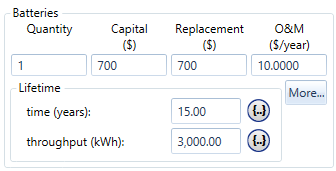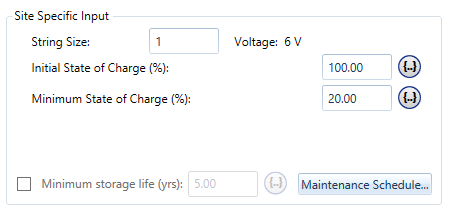# HOMER Pro 3.14

 Navigation: Design > Components Tab > Storage SupercapacitorThe Supercapacitor is based on the Idealized Storage Model. The Idealized Storage Model replicates a simple storage model that assumes a flat discharge curve because the supply voltage stays mostly constant during the discharge cycle. For this model, you need to enter only the nominal capacity in amp-hours. HOMER uses this as the actual capacity of the storage. Some high performance Lithium Ion batteries, for example, can be modeled well with an Idealized Storage model. To learn more about this model, refer the article on the Idealized Storage Model.

## Properties of the Supercapacitor

This section contains information about the nominal voltage, nominal capacity, and maximum charge and discharge current of the idealized battery. The Energy (joules) stored in a supercapacitor can be calculated using the following formula:

Ejoules = 1/2 C V2 (1)

In the equation above, E is the energy stored in joules, C is the capacitance in farads, and V is the voltage. You can specify the nominal capacity as the energy capacity (in watt-hours) divided by the nominal voltage, to get an effective capacity in amp-hours. This is not the same as calculating the charge capacity of a capacitor (farads x volts), which produces the correct total energy capacity. Equation (1) above takes into account the proportional decrease in voltage over the discharge of a capacitor. The calculation for the nominal capacity input (in Ah) for HOMER is as follows:

NAh = Ejoules / V / 3600 = 1/2 C V / 3600 (2)

In this equation, NAh is the nominal capacity in amp-hours. You divide by 3600 to convert from joules to watt hours (or, equivalently, from coulombs or amp-seconds to amp-hours).